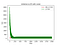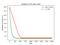# L1 loss, abs L1 loss, and L2 loss

We are commonly using L2 loss in deep learning. My question is why not use L1 loss? Here, I’d like to do an experiment to explain.

## Experiment setup

wx = y

where we only have one parameter which is w and in order to make the problem even simple, we’d like also fix the value of x by picking up a value of 2 for x.

So what we are going to estimate is

2*w = y

For the regular wx+b=y estimation, please check this code.

we set the unknow w as 1 and we are going to estimate this w.

## prepare the data

`# simulate the l1 loss and l2 loss# simulate w*x = y where x is a fixed number 2# It means we are going to simulate 2*w = y# a noise item will be added which is z. # 2*w + z = y# if z is 0, then there is no noise, # else some gaussian noise will be addedimport numpy as npimport randomimport matplotlib.pyplot as plt# number of samplesN = 20INIT_w = 20# our target w is 1.0add_noise = Falsex = 2*np.ones((N,))if add_noise:    z = np.random.normal(0, 0.05, N)    y = 1.0*x + zelse:    y = 1.0*x`

## Define the loss

`def loss(y_hat, y, loss='L1'):    if loss=='L1':        print('L1 loss')        return  np.sum(y_hat - y)    elif loss == 'L2':        print('L2 loss')        return np.sum(np.square(y_hat-y))`

## Train the model using a gradient descent algorithm

`epoches = 20batch_size = 2batch_start_idx = list(range(0, N, batch_size))l1_losses, l2_losses = [], []w_l1_loss, w_l2_loss = [], []# set learning rate as 1.0lr = 0.01for loss_type in ['L1', 'L2']:    w = INIT_w     for epoch in range(epoches):        for batch_i in batch_start_idx:            this_x, this_y = x[batch_i:batch_i+batch_size], y[batch_i:batch_i+batch_size]            y_hat = w*this_x            L = loss(y_hat, this_y, loss_type)            if loss_type == 'L1':                l1_losses.append(L)                print(f"{loss_type}, {L}")                # L = y_hat -y , dL/dy_hat = 1                # dy_hat/dw = x                # dL/dw = 1*x                gradient = np.sum(x)                print(gradient)                w -= lr*gradient                w_l1_loss.append(w)            elif loss_type == 'L2':                l2_losses.append(L)                print(f"{loss_type}, {L}")                # L = (y_hat -y)**2 , dL/dy_hat = 2(y_hat - y)                # dy_hat/dw = x                # dL/dw = 2(y_hat-y)*x                gradient = np.sum(2*(y_hat-this_y)*this_x)                w -= lr*gradient                w_l2_loss.append(w)`

## Visualization of the algorithm

`plt.plot(range(len(l1_losses)), l1_losses, c = 'r', label='L1 loss')plt.plot(range(len(l2_losses)), l2_losses, c = 'g', label='L2 loss')plt.xlabel('step')plt.ylabel('loss')if add_noise:    plt.title('initialize w:'+str(INIT_w)+' with noise')else:    plt.title('initialize w:'+str(INIT_w)+' without noise')plt.legend(loc='best')if add_noise:    plt.savefig('loss_l1_l2_with_noise.png')else:    plt.savefig('loss_l1_l2_without_noise.png')plt.show()plt.close()plt.plot(range(len(w_l1_loss)), w_l1_loss, c = 'r', label='L1 loss')plt.plot(range(len(w_l2_loss)), w_l2_loss, c = 'g', label='L2 loss')plt.xlabel('step')plt.ylabel('estimate w (target is 1)')if add_noise:    plt.title('initialize w:'+str(INIT_w)+' with noise')else:    plt.title('initialize w:'+str(INIT_w)+' without noise')plt.legend(loc='best')if add_noise:    plt.savefig('w_l1_l2_with_noise.png')else:    plt.savefig('w_l1_l2_without_noise.png')plt.show()`

## Visualization results

From the second plot, we can see by using L1 loss, the estimation fails as the gradient descent algorithm will push the loss function go to a smaller value. So by using L1 loss, it fails. If we keep on training, the w will go to -inf.

## What if we change the L1 loss to abs L1 loss?

When sigma is small which is 0.05, we have

When sigma is large say sigma = 5Loss is not stable if we use L2 loss as L2 loss is more sensitive to noiseThe estimation based on L2 loss is better.

The estimation of L2 is better than based on abs L1 loss. We believe it is because the L2 gradient will be reduced if it is close to the global minimum. However, for the abs L1 the magnitude of the gradient keeps the same.

## How to address this issue?

current learning rate = 0.001

change it to 0.0001

We need more iterations in this case and the results of abs L1 loss is better than before.

Also we can use an exponential decay learning rate.

we reset the learning rate to 0.01 and decay = 1/2000

`lr = init_lr*(1. / (1. + decay * iterations))`

When the learning rate is small, the updating process will be dominated by the gradient. The gradient will have fluctuations with the noise. This explains why the update of w has fluctuated.